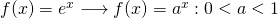# Sets Identity function

The function that associates each real number to it self is called the identity function and denoted by I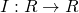I(x) = x : x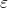R

The domain and range of the identity function are both equal to R. the graph is a straight line passing through the origin and inclined at an angle of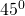with x axis
F(x) = x
F(1) = 1
F(2) = 2
Modulus Functions:-
If f(x) = |x| = {x when x ≥ 0,
-x when x < 0} Is called the modulus function or absolute value function the domain of the modulus function is the set of all real number. & range is the set of all non negative real number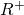= {xR : x ≥ 03}
(1) If x ≥ 0 the graph coincide. With the graph of
the identity functional (g= x)

If x < 0 it is coincide to the line y = -x

Properties of Modulus Function

1) For any real number x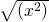= |x|

2) a,b is a positive real number, then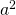|x| ≤ a -a ≤ x ≤ a|x| ≥ a x ≤ -a or x ≥ a<|x| < a -a < x < a>|x| > a x < -a or x > a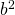a≤ |x| ≤ b x[-b, -a] u [a,b]<<a ≤ |x| ≤ b[-b, -a] u [a,b]

3) for real number x and y

|x+y| = |x| + |y| (x≥0 and y≥ 0) or (x< 0 and y< 0)|x-y| = |x| - |y| (x≥0 and |x|≥ |y|) or (x≤ 0 , y≤ 0 and |x| ≥ |y|)|x± y| ≤ |x| + |y||x± y| ≥ |x| - |y|

Greatest Integer Function (Floor Function)
f(x) = [x] for all xεR or ⌊x⌋ is called greatest integer function

for any real number x the smallest [x] to denote the greatest integer less than or equal to x
Domain of the greatest integer function is the set of R to all real number and the range is the set fo Z of all integers as it’s attains only integer value.

e.g. [2.75] = 2, = 3, [0.74] = 0, [-7.45] = -8 etc

Properties :- if n is integer and x is a real number between n and n+1 then

[-n] = -[n]
[x + k] = [x] + k for any integer k
[-x] = -[x] -1

[x] + [-x] =[x] – [-x] =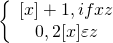[x] ≥ k = x ≥ k where kz
[x] ≤ k = x < k where kz
[x] > k = x ≥ k +1 where kz
[x] < k = x < k where kz
[x+y] = [x] + [y+x-[x] for all x,yR= [n x], nN

Smallest Integer Function
f(x) = ⌈x⌉ for all x εR
Is called the smallest integer function or the ceiling function.

The domain of the smallest integer function is the set of R of all real number and its range is the set z 0 < all integers e.g :- ⌈4.75⌉=5, ⌈-7.2⌉ = -7,⌈5⌉=5, ⌈.75⌉=1 etcProperties of Smallest Integer Function ⌈-n⌉= -⌈n⌉ ,nz

⌈-x⌉= -⌈x⌉+ 1,xR-z

⌈x+n⌉= ⌈x⌉+ n,xεR-z and nz
⌈x⌉+ ⌈-x⌉ =⌈x⌉- ⌈-x⌉ =FRACTIONAL PART FUNCTION

The function f(x) = {x} for all xεR
The symbol {x} denote the fractional part of or decimal part of x.
The domain of the fractional part function is the set of R of real number and range is the set [0,1]
f(x) = {x} = x – [x] : x εR
e.g. 3.45 = 0.45, [2.75] = 0.25, [-0.55] = 0.45,  = 0, [-7] = 0 etc

SIGNUM FUNCTION

If f(x) =.3 × .3 =.09
f(x) =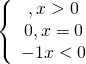The domain of the signum function is the set of R (all real number) and the domain is the set
(-1,0,1)

EXPONENTIAL FUNCTION:-
F (x) =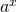where a> 0 and a ≠ 1
If a > 1
If y = f(x) =We observe that –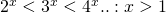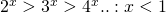If 0 < a<1Y = f(x) =decrease with the increase in x.
And y>0 : x ε Rif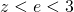then graph